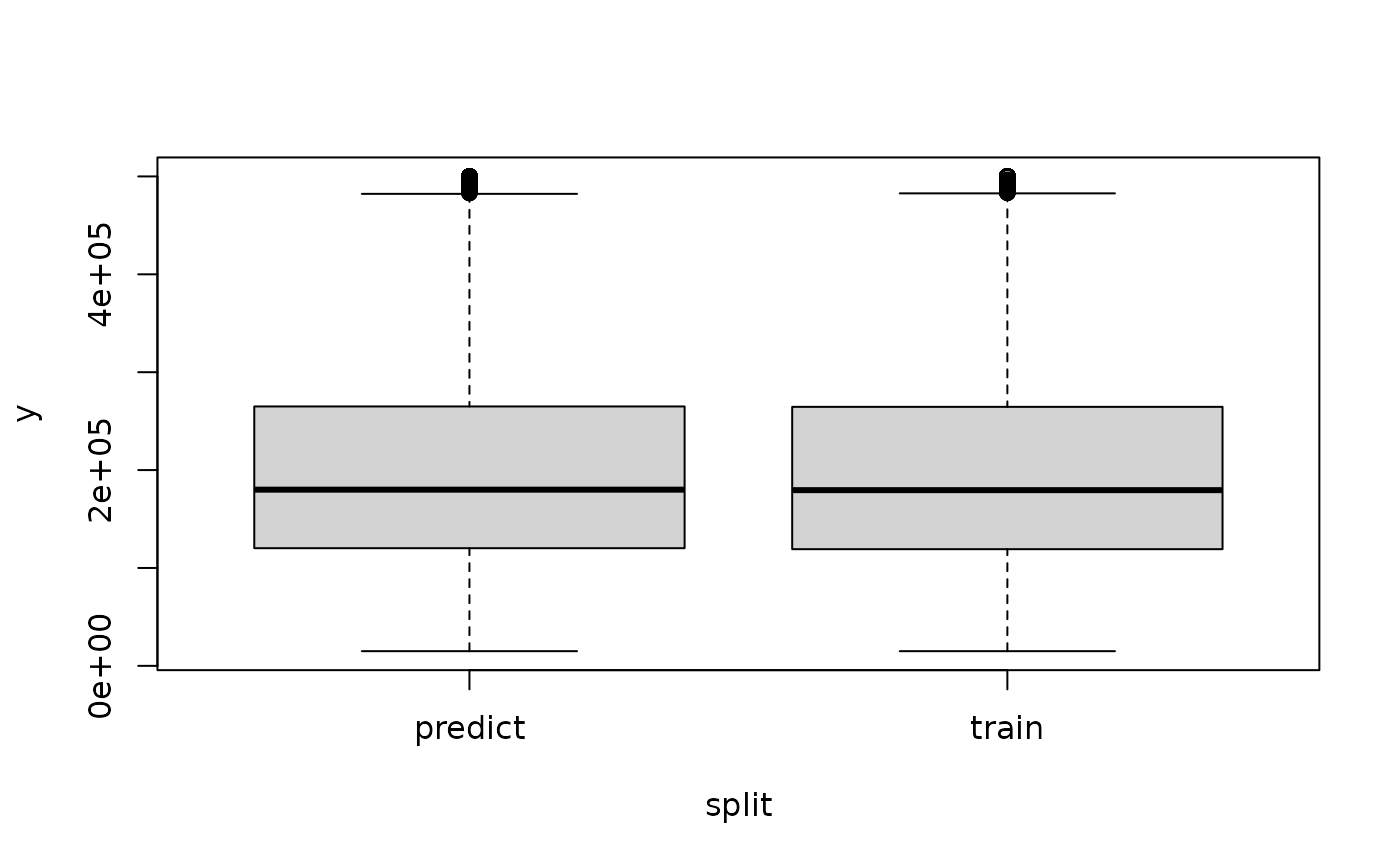Creates a split of the row ids of a Task into a training set and a test set while optionally stratifying on the target column.

For more complex partitions, see the example.

## Usage

partition(task, ratio = 0.67, stratify = TRUE, ...)

partition(task, ratio = 0.67, stratify = TRUE, bins = 3L, ...)

partition(task, ratio = 0.67, stratify = TRUE, ...)

## Arguments

ratio

(numeric(1))
Ratio of observations to put into the training set.

stratify

(logical(1))
If TRUE, stratify on the target variable. For regression tasks, the target variable is first cut into bins bins. See Task$add_strata(). ... (any) Additional arguments, currently not used. bins (integer(1)) Number of bins to cut the target variable into for stratification. ## Examples # regression task task = tsk("boston_housing") # roughly equal size split while stratifying on the binned response split = partition(task, ratio = 0.5) data = data.frame( y = c(task$truth(split$train), task$truth(split$test)), split = rep(c("train", "predict"), lengths(split)) ) boxplot(y ~ split, data = data)# classification task task = tsk("pima") split = partition(task) # roughly same distribution of the target label prop.table(table(task$truth()))
#>
#>       pos       neg
#> 0.3489583 0.6510417
prop.table(table(task$truth(split$train)))
#>
#>       pos       neg
#> 0.3495146 0.6504854
prop.table(table(task$truth(split$test)))
#>
#>       pos       neg
#> 0.3478261 0.6521739

# splitting into 3 disjunct sets, using ResamplingCV and stratification
task$set_col_roles(task$target_names, add_to = "stratum")
r = rsmp("cv", folds = 3)$instantiate(task) sets = lapply(1:3, r$train_set)